David Tong: Cambridge Lecture Notes on Theoretical Physics

# David Tong: Lectures on Theoretical Physics

## Classical Mechanics## Dynamics and Relativity

An introduction to Newtonian Mechanics and Special Relativity, aimed at first year undergraduates.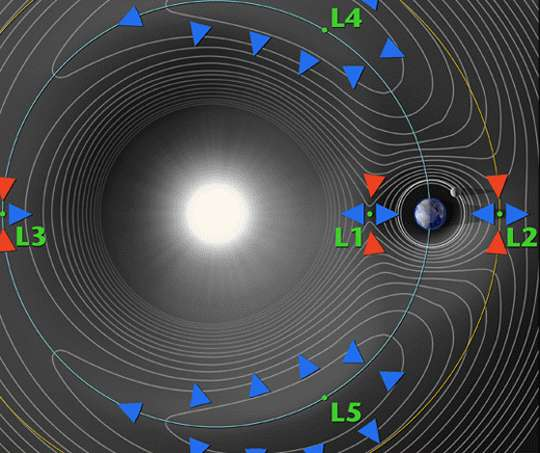## Classical Dynamics

A second course on classical mechanics, covering the Lagrangian and Hamiltonian approaches, together with a detailed discussion of rigid body motion.

## Electromagnetism## Vector Calculus

A course for first year undergraduates, describing integral theorems and all things ∇.## Electromagnetism

A course on electromagnetism, starting from the Maxwell equations and describing their application to electrostatics, magnetostatics, induction, light and radiation. The course also covers the relativistic form of the equations and electromagnetism in materials.

## Quantum Mechanics## Quantum Mechanics

A first course on quantum mechanics, focussing mostly on the Schrödinger equation.## Topics in Quantum Mechanics

An advanced course on quantum mechanics, aimed at final year undergraduates. It covers atomic physics, scattering theory, quantum foundations and a number of other topics.

## Condensed Matter Physics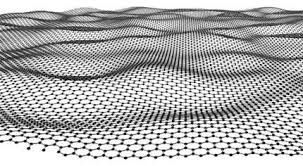## Solid State Physics

An introductory course on solid state physics, aimed at final year undergraduates. It cover the basics of band theory, Fermi surfaces, phonons and particles in magnetic fields.## Quantum Hall Effect

An introduction to the quantum Hall effect. The first half uses only quantum mechanics and is at a level suitable for undergraduates. The second half covers more advanced field theoretic techniques of Chern-Simons and conformal field theories.

## Statistical Physics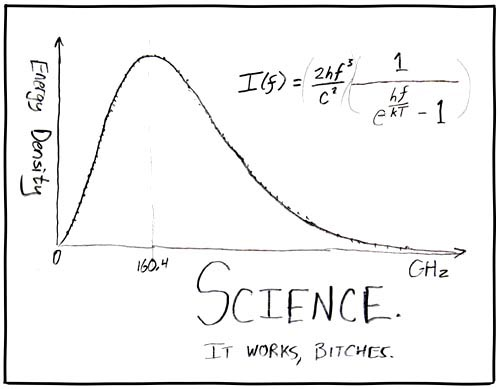## Statistical Physics

An introduction to statistical mechanics and thermodynamics, aimed at final year undergraduates. After developing the fundamentals of the subject, the course covers classical gases, quantum gases and phase transitions.## Kinetic Theory

A graduate course on basic topics in non-equilibrium statistical mechanics. It covers kinetic theory and the Boltzmann equation, stochastic processs and linear response.## Statistical Field Theory

An introductory course on phase transitions and critical phenomena, aimed at first year graduate students. The main goal is to get to grips with the renormalisation group.

## Gravitation and Cosmology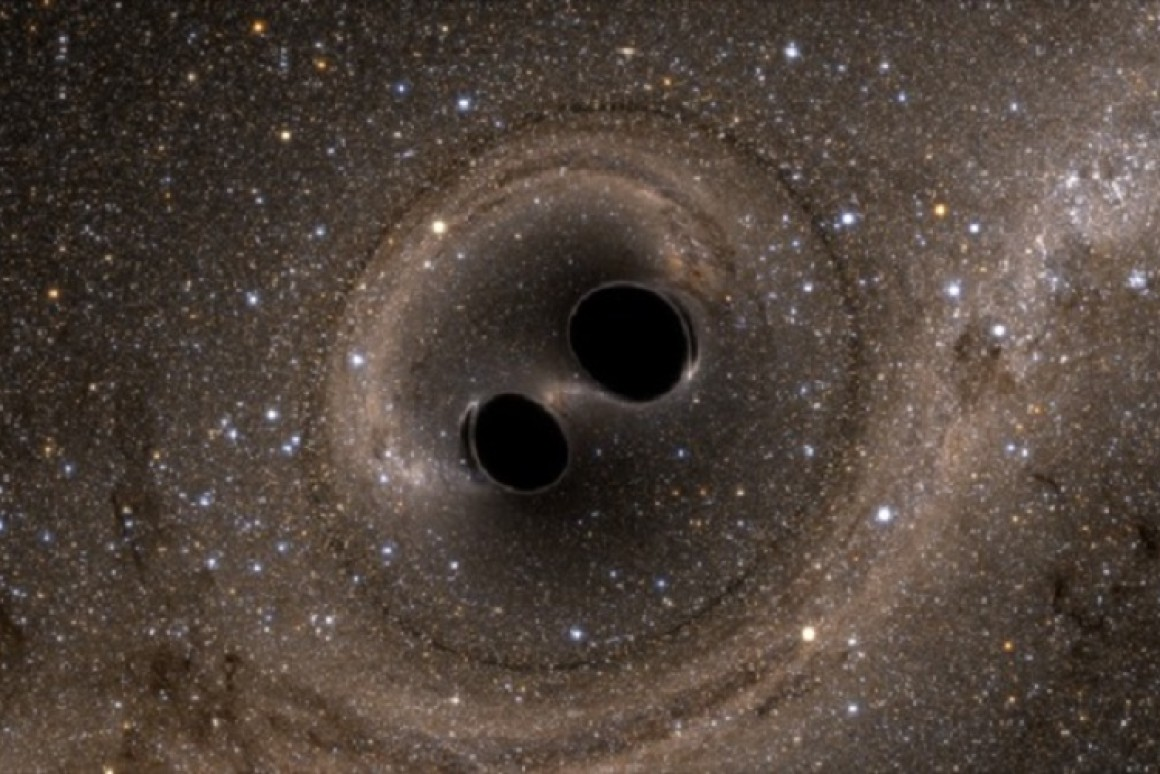## General Relativity

An introduction to general relativity, aimed at first year graduate students. It starts with a gentle introduction to geodesics in curved spacetime. The course then describes the basics of differential geometry before turning to more advanced topics in gravitation.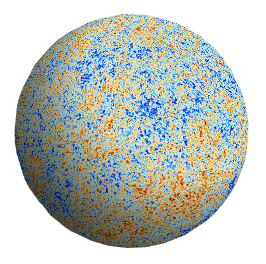## Cosmology

An introductory course on cosmology, aimed at final year undergraduates. It covers the expanding universe, thermal history, and structure formation.

## Quantum Field Theory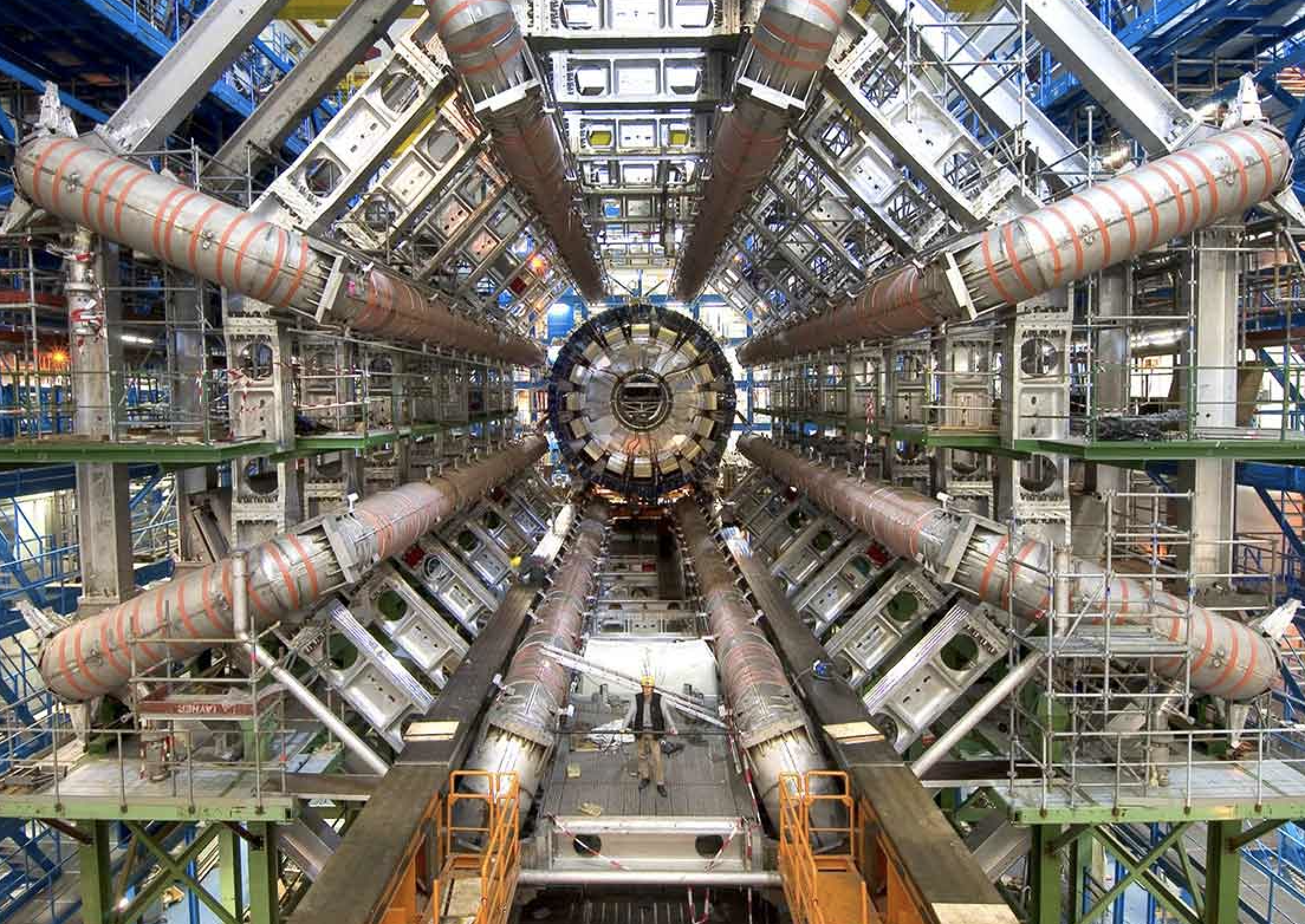## Particle Physics

An elementary course on elementary particles. This is, by some margin, the least mathematically sophisticated of all my lecture notes, requiring little more than high school mathematics. The lectures provide a pop-science, but detailed, account of particle physics and quantum field theory.## Quantum Field Theory

An introductory course on quantum field theory, aimed at first year graduate students. It covers the canonical quantization of scalar, Dirac and vector fields. Videos are also included.## Gauge Theory

These notes provide an introduction to the fun bits of quantum field theory, in particular those topics related to topology and strong coupling. They are aimed at beginning graduate students and assume a familiarity with the path integral.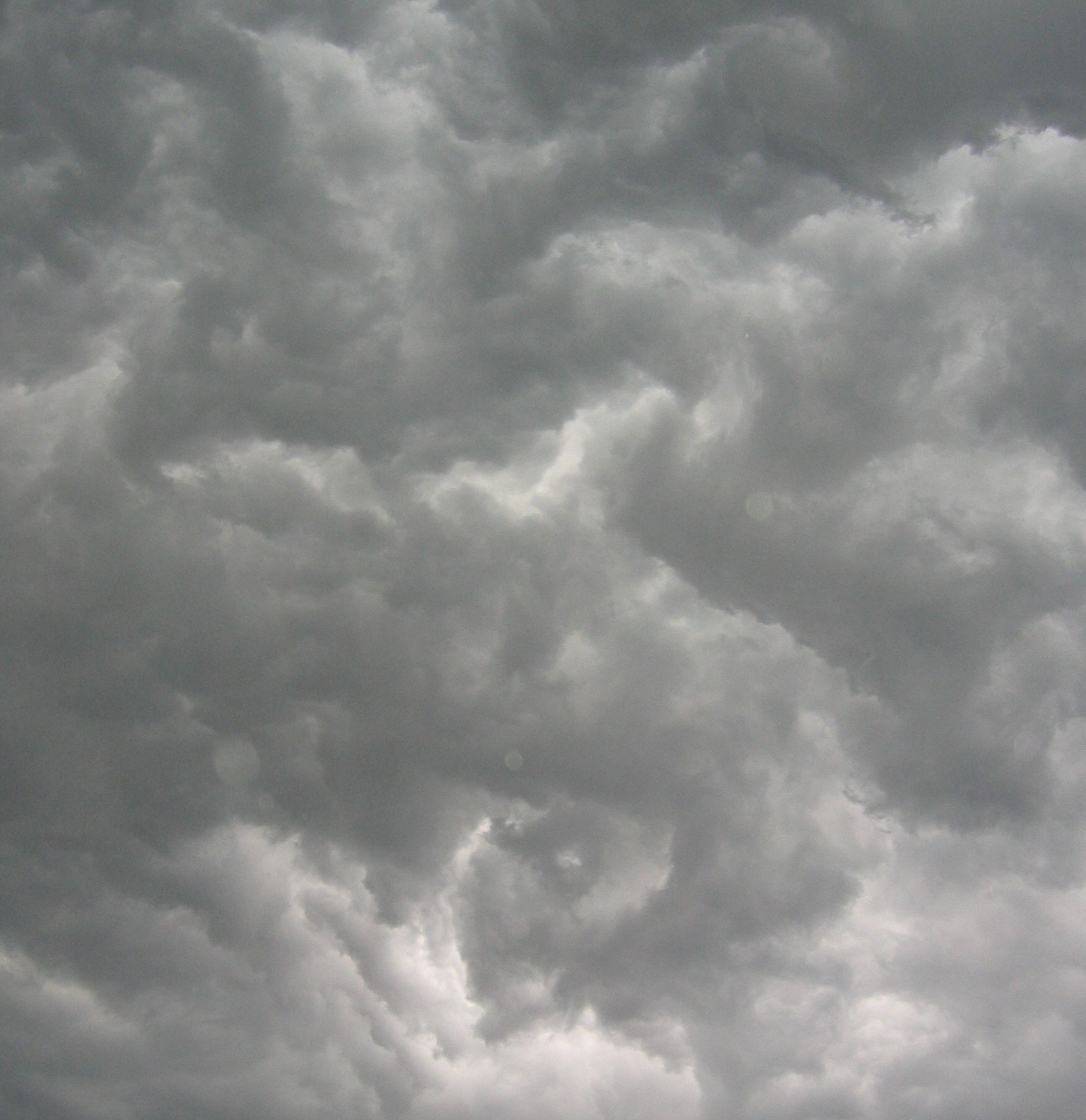## Solitons

A course for advanced graduates students, covering solitons in supersymmetric gauge theories and D-branes. It was given at the TASI summer school.

## String Theory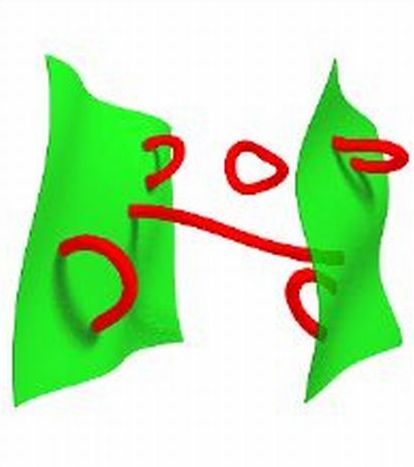## String Theory

An introduction to string theory, aimed at first year graduate students. It covers the bosonic string and the basics of conformal field theory.